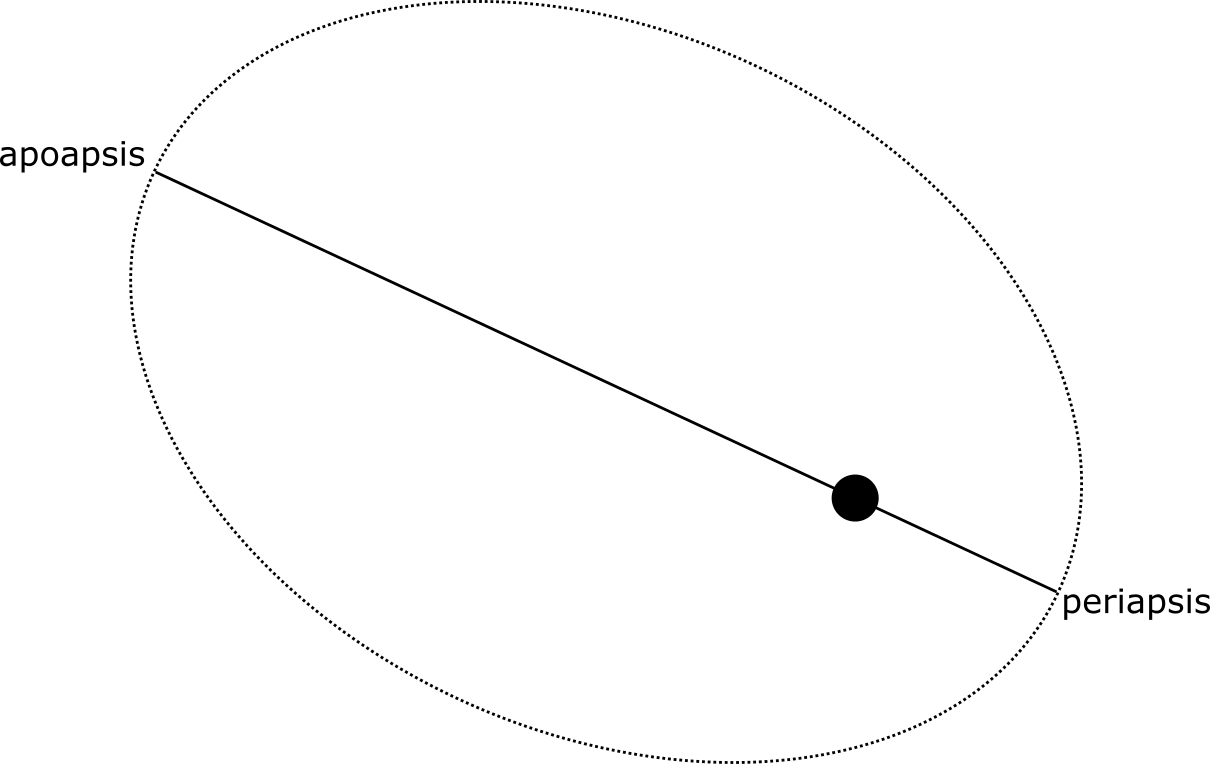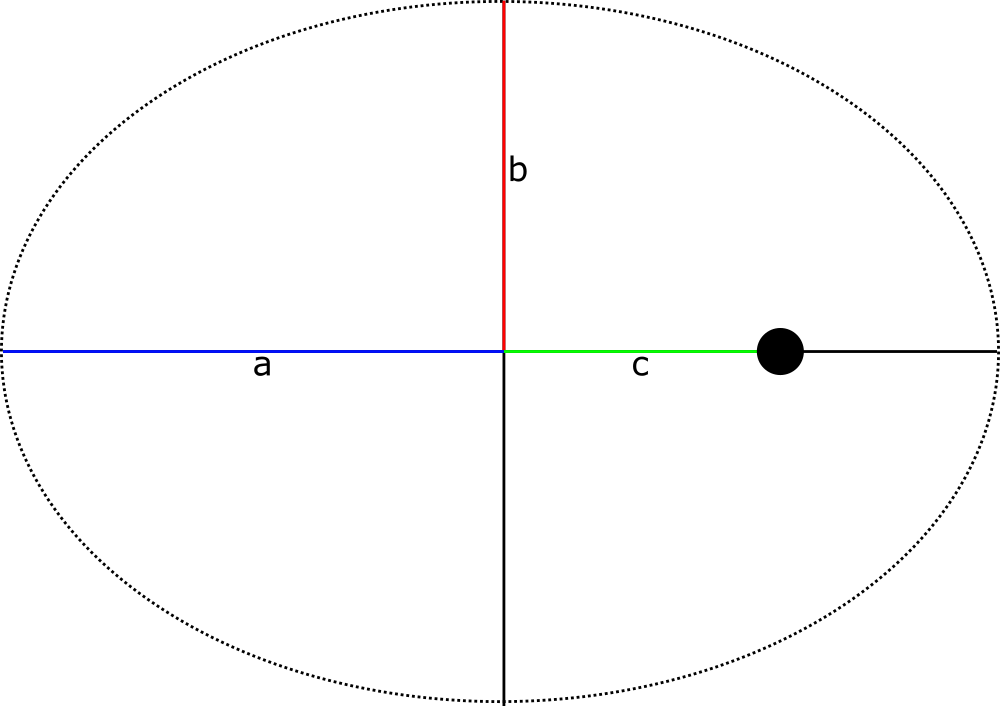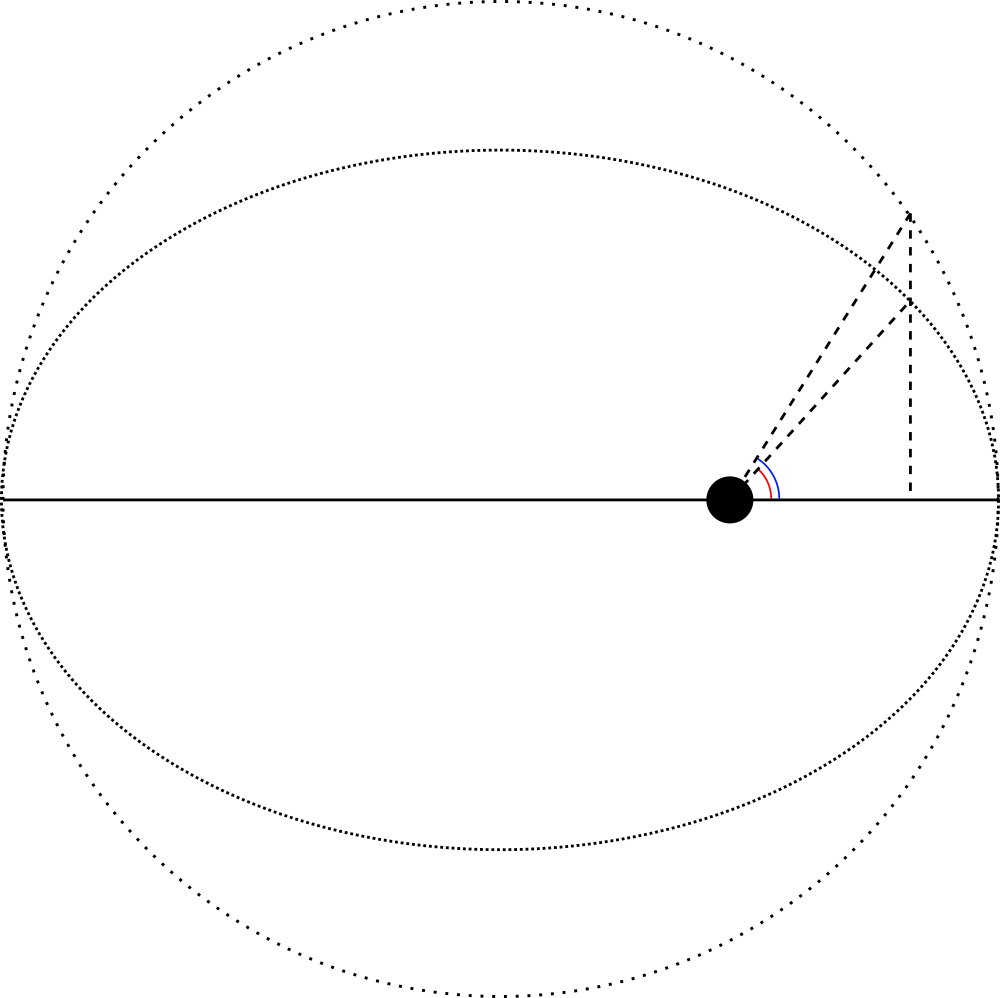# The math behind elliptical orbits

/ game physics

## Introduction

Coincidences rarely happen in physics, and perfectly circular orbits statistically don't exist.

The centripetal force will either be larger or smaller than the gravitational force at any given point in the orbit. This is what leads to elliptical orbits. When the centripetal force is larger, the satellite will start moving away from the primary. As the satellite does so, the velocity vector will no longer be orthogonal to the gravitational acceleration. Consequently, the satellite starts losing speed and the centripetal force gets less. Since the satellite is moving further away, the gravitational force also becomes less. If the satellite has too much energy, the gravitational force may never be enough to outweigh the centripetal force, which causes a hyperbolic trajectory. However, if the satellite lose enough energy for the gravitational force to become the strongest, the satellite will start falling back to the primary. This is when the opposite process happens, as gravity causes the satellite's velocity to increase, and the process repeats. We have our elliptical orbit.The point where the satellite is closest to the primary is called the periapsis. This is the point where the satellite has the most energy. The point in the orbit where the satellite is furthest from the primary is called the apoapsis, and this is where the energy of the satellite is the lowest. You may have heard terms such as apohelion and perigee before. Most known bodies have there own suffixes for the highest and lowest points of orbits around them. Apogee and perigee are the highest and lowest points in an orbit around Earth, apohelion and perihelion the highest and lowest points in an orbit around the Sun.

## Ellipses

It is no surprise that the equations for elliptical orbits are more complex than the orbital ones. As you may have guessed, an elliptical orbit is an ellipse where the primary is one of the foci. To understand elliptical orbits, we need to understand ellipses first.

Just as the circular orbits are described by a circle around its center, elliptical orbits are described by an ellipse around two foci. In a circle each point has the same distance to the center; in an ellipse each point yields the same result if you add up the distances to the two foci (sg. focus). The line through the two foci is called the semi-major axis, and the line through the center of the ellipse orthogonal to the semi-major axis is the semi-minor axis. The distance from the center to the ellipse along the semi-major and semi-minor axis are denoted by $a$ and $b$ respectively. See the following image for a representation of these concepts:The distance from the center to one of the foci $c$ is called the linear eccentricity. If you divide this by the distance $a$, you get the eccentricity of the ellipse, which describes how stretched the ellipse is. Eccentricity is a commonly used term to describe orbits. As the orbit approaches a circular orbit more and more, the focus will come closer to the center of the ellipse, and the eccentricity will tend towards 0.

For orbits, we are often not given the values $a$ and $b$, but the apoapsis and periapsis heights. However, as you can see, if you add up apoapsis and periapsis heights, you should get exactly $2a$. We also know the centre of the ellipse (it's exactly between apoapsis and periapsis), and thus we can calculate the distance between the centre and the primary to obtain $c$. We now have $a$ and $c$ which together allow us to calculate the eccentricity $e$, and now we have all we need to start expressing this orbit using the power of math!

## Position

Remember that for circular orbits, we were able to determine the position over the parameter $\theta$ as:

$$\begin{pmatrix}x \ y\end{pmatrix} = r \begin{pmatrix}\cos \theta \ \sin \theta\end{pmatrix}.$$

For elliptical orbits, we can do something very similar:

$$\begin{pmatrix}x \ y\end{pmatrix} = \begin{pmatrix}a \cos E \ b \sin E\end{pmatrix}.$$

Instead of using a single radius $r$, we use $a$ and $b$ instead to represent that the ellipse has a different size horizontally as vertically. I also replaced the parameter $\theta$ with $E$. As opposed to what you may think, $E$ is not actually the angle between the semi-major axis and the line through the center of the ellipse and the point on the ellipse.

Since circles are easier to reason about, we use a circle to help us with ellipses. We create a circle which shares a centre with the ellipse, and has radius $a$ so it touches the ellipse in its two furthest points.The angle $E$ we are talking about is the angle between the center of the ellipse and the vertical projection of the point on the ellipse to this circle (blue in the image above), and is called the eccentric anomaly. The actual angle between the point on the ellipse and the semi-major axis (red in the image above) is called the true anomaly and often denoted by $\nu$.

## Velocity

We can now draw an orbit, but we still haven't figured out the velocity of the satellite at any point in the orbit. Unlike circular orbits, the orbital speed of the satellite will change over time. This is where it gets a bit tricky. In circular orbits, the satellite moves at a constant rate, so we can increase $\theta$ at a constant rate, but for elliptical orbits we need to do more work to express $E$ as a function of time passed.

We can find the velocity $v$ of an object at any point in the orbit given $E$, but this isn't enough to give us the rate of change of $E$. We could use this to approximate the rate of change of $E$ by dividing among the radius of the auxilary circle to get the angular velocity. This may be a close enough approximation for a lot of applications.

For the velocity we have the following equation:

$$\tan{\frac{v}{2}} = \sqrt{\frac{1 + e}{1 - e}}\tan{\frac{E}{2}}.$$

For an approximate velocity of $E$, we'd find:

$$\tan{(\pi a \dot{E})} \approx \sqrt{\frac{1 + e}{1 - e}}\tan{\frac{E}{2}}.$$

Or in a direct expression:

$$\dot{E} \approx \frac{\arctan{\left(\sqrt{\frac{1 + e}{1 - e}}\tan{\frac{E}{2}}\right)}}{2 \pi a}.$$

Here all variables are as before, and $e$ is the eccentricity of the ellipse.

Of course as this is an approximation it is prone to artefacts. For relatively small $e$ and most use cases in, say, games, this may be acceptable if the update steps are small. If we want to make sure that the orbit takes the correct amount of time though, we have to go a step further and introduce the concept of mean motion $n$. The mean motion is simply the average change of $E$ over time. This is simply $2 \pi$ divided by the period of the orbit.

Now we have all the concepts to build Kepler's equation:

$$E - e \sin{E} = (t - t_\text{per}) \cdot n.$$

In this equation, $t_\text{per}$ is the time at which the satellite passes through the periapsis of the orbit.

There we have it. For any given point in time $t$ we can find the eccentric anomaly $E$, convert that into the position, and we're done. Sadly, solving Kepler's equation isn't a thing we can easily do, as there isn't a so-called closed-form solution, which would mean we could express $E$ as some expression as we did with the approximation earlier. There are several ways around that, which we will explore in the next post, where we will also be looking at how to go about writing the code for elliptical orbits.